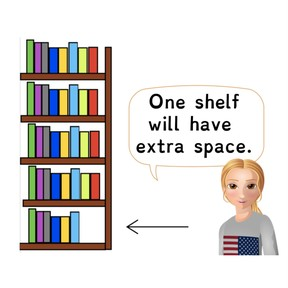Interpreting the remainder of a division problem

# Interpreting the remainder of a division problem

I can solve a story problem using division and interpret the remainder.8,000 schools use Gynzy92,000 teachers use Gynzy1,600,000 students use Gynzy

## General

In this lesson, students will solve several story problems in which there is a remainder and decide how the remainder changes the answer. For example, will you round up? Or will you add one more to the total? They will learn different strategies and ways to interpret the remainder by thinking about what it stands for. They will be able to recognize when to drop the remainder, share it, write it as a fraction, use it as the answer, or add one more to the quotient.

## Standards

CCSS.MATH.CONTENT.4.OA.A.3

## Learning Objective

Students will be able to solve a word problem that contains a remainder and determine how the remainder will affect the final answer.

## Introduction

Begin by explaining that remainders are like “leftovers.” Ask students what they do with leftover items like food or paint. How do they make that decision? After this, review some basic facts and explain that division problems can be used to show the distribution of items and sharing. However, when sharing, there is sometimes a remainder. Demonstrate this to students by having them drag the strawberries to the slices of cakes. There will be 2 strawberries leftover.

## Instruction

Explain and model what it means to interpret the remainder of a division problem using the examples provided. Students can drop the remainder when there are extras they don’t really need. The remainder can also be shared. For example, if there is a remainder of \$1, you can share it by giving 50 cents to each person. Another way to interpret a remainder is to write it as a fraction. This is done when measuring. You can also add one more to the quotient. This can be done in a situation where someone will be left out. Finally, the remainder is sometimes the answer to the story problem, so be sure to read carefully! Have students practice the problems on the board. Students should also explain how they interpreted the remainders in their answers and why.

## Quiz

Students respond to ten multiple-choice and fill-in responses in which students calculate and interpret the remainder of a problem.

## Closing

Students will work together to plan a field trip! They will split into groups and each group will be assigned a committee: Transportation, food, activities, and cost. Each group will work together to create a story problem for their committee in which there is a remainder and the rest of the class will solve it.

### The online teaching platform for interactive whiteboards and displays in schools

• Save time building lessons

• Manage the classroom more efficiently

• Increase student engagement